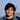# Point free programming and function composition

Point free programming (a.k.a. Tacit programming), formally, is a programming paradigm in which function definitions don’t include information about their arguments. It instead focuses more on combinators and composition to manipulate the arguments themselves.

In simpler terms, point free programming focuses on eliminating unnecessary parameters and arguments from the code. The term point refers to a function’s parameter input. This methodology is also sometimes referred to as equational reasoning in more functional languages. I think equational reasoning is a means to make a program point free.

Here’s an example of making code point free:

``````const add1 = n => n + 1;

[1, 2, 3].map(n => add1(n)); // [2, 3, 4]

// A point free variant - unnecessary parameter eliminated
[1, 2, 3].map(add1); // [2, 3, 4]``````

Point free style can also be combined with currying (previous blog post).

``````const add = (a, b) => a + b;

[1, 2, 3].map(curry(add)(1)); // [2, 3, 4]``````

Here, the first parameter (value to be incremented by i.e. `1`) was partially applied. Later when mapping over the array the second parameter was passed to the curried function.

## Point free utilities

You may adopt point free style for any function depending on the usecase. I’ve noted down a few utilities to get started with. Most of these are available in functional libraries such as RamdaJS.

### not or complement

Negates a function.

``````const not = fn => (...args) => !fn(...args);

const isEven = n => n % 2 === 0;
const isOdd = not(isEven);

console.log(isEven(4)); // true
console.log(isOdd(5)); // true``````

### when

Takes a predicate (a function that returns a boolean) and a function as input. The function is executed and the result returned if the predicate evaluates to `true`.

``````const when = (predicate, fn) => (...args) => predicate(...args) && fn(...args);

// A predicate
const isEven = n => n % 2 === 0;

// A function
const logger = value => console.log(value);

when(isEven, logger)(2); // 2
when(isEven, logger)(4); // 4``````

## Composition

Composition refers to using functions together. When combining functions, the order of composition matters. For example, consider the example from the first blog post.

``````const add1 = num => num + 1;
const mul2 = num => num * 2;

// compose a new function using the above two
// note the order of function invocation matters

Although we read the composition from left to right, the actual order of function invocation happens right to left, i.e. first `add1` is invoked and then the result is passed to `mul2`.

A couple of useful utilities for composition are `compose` and `pipe`.

### compose

Returns a composed function from the inputs which executes right to left (as displayed in the example above).

``````const compose = (...fns) => arg =>
[...fns].reverse().reduce((result, fn) => fn(result), arg);

const add1 = num => num + 1;
const mul2 = num => num * 2;

mul2,
); // right to left order

### pipe

Pipe is similar to `compose` but follows a more natural order of left to right invocation of composed functions.

``````const pipe = (...fns) => arg =>
[...fns].reduce((result, fn) => fn(result), arg);

const add1 = num => num + 1;
const mul2 = num => num * 2;

mul2,
); // left to right order